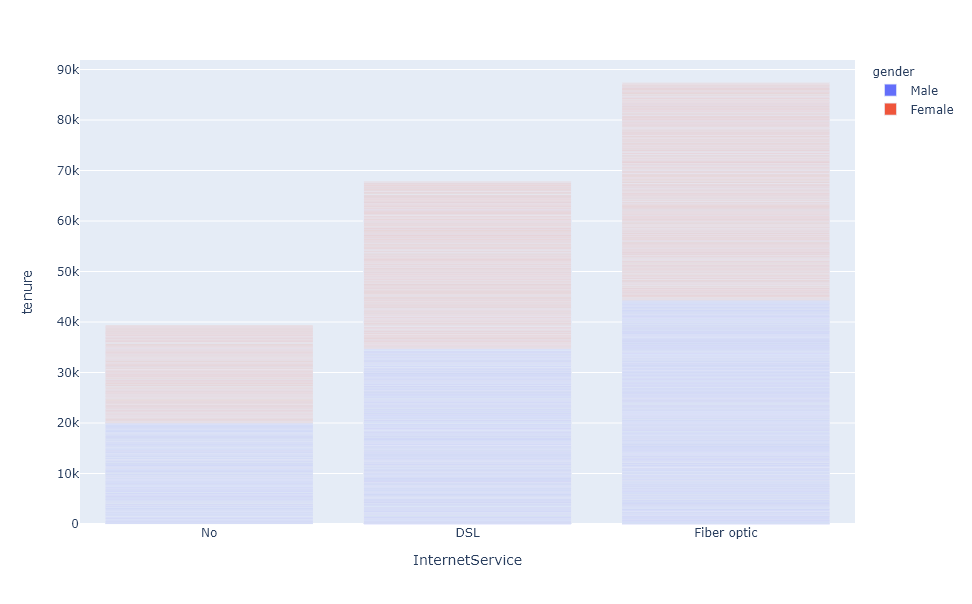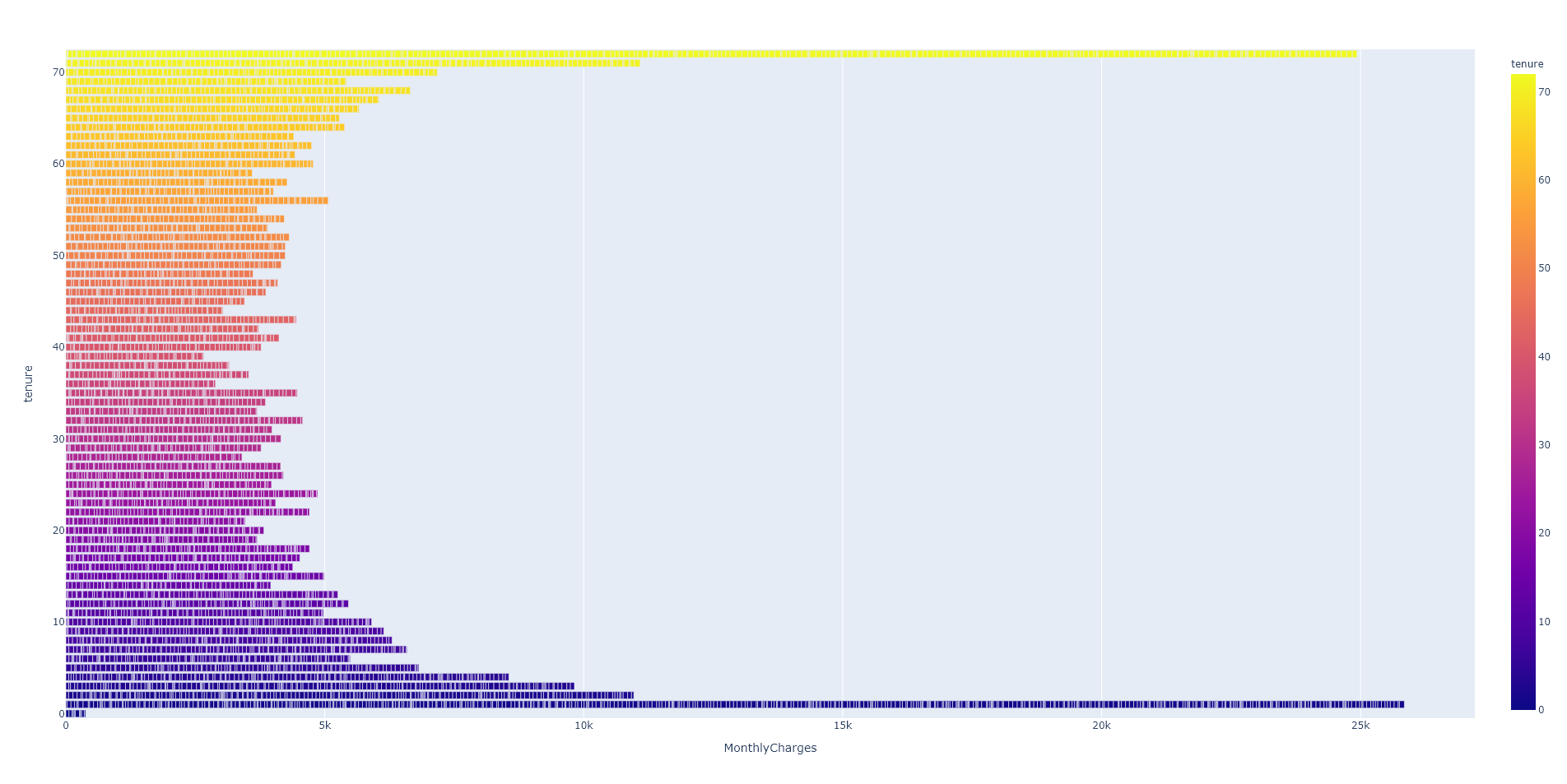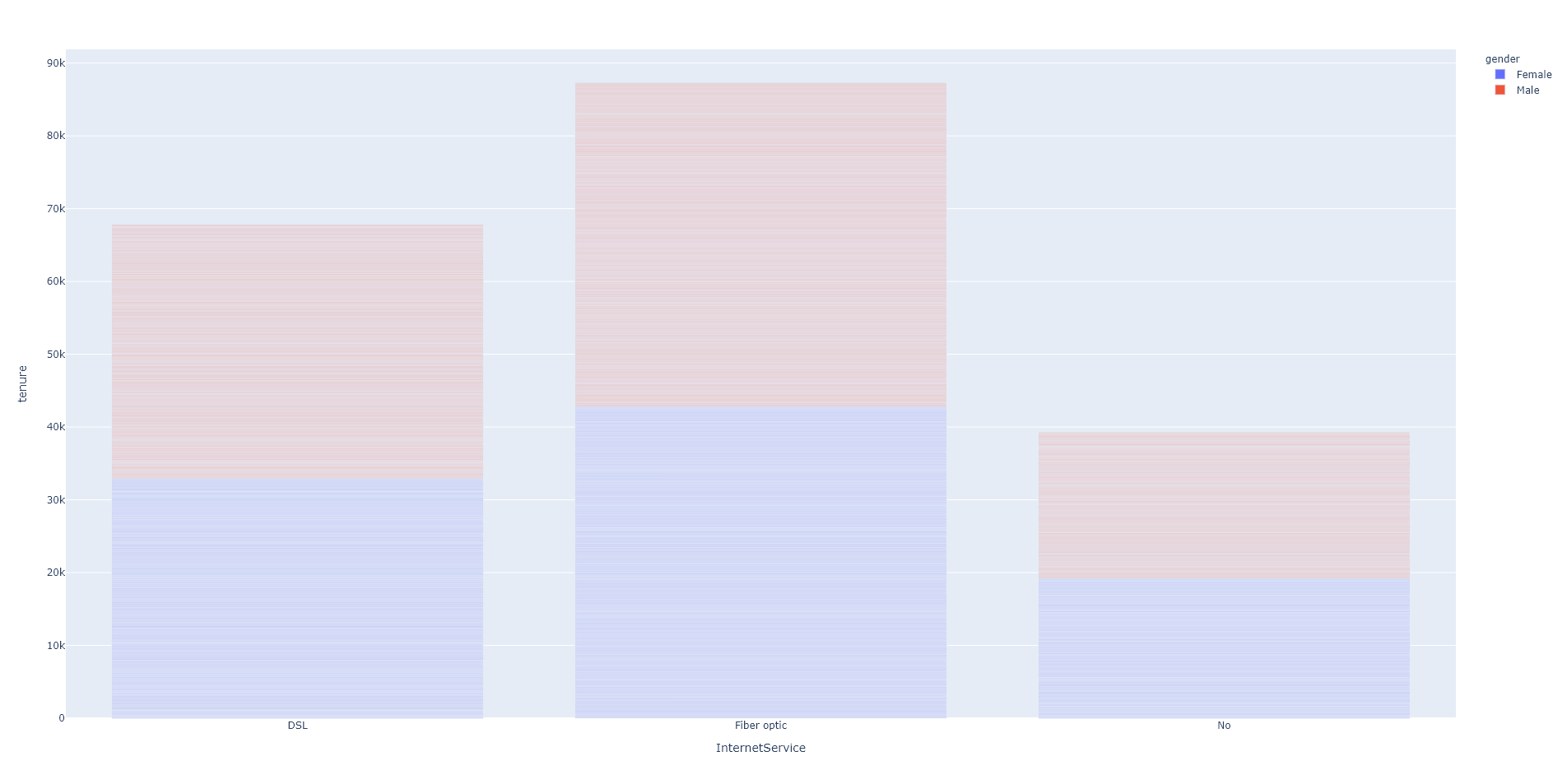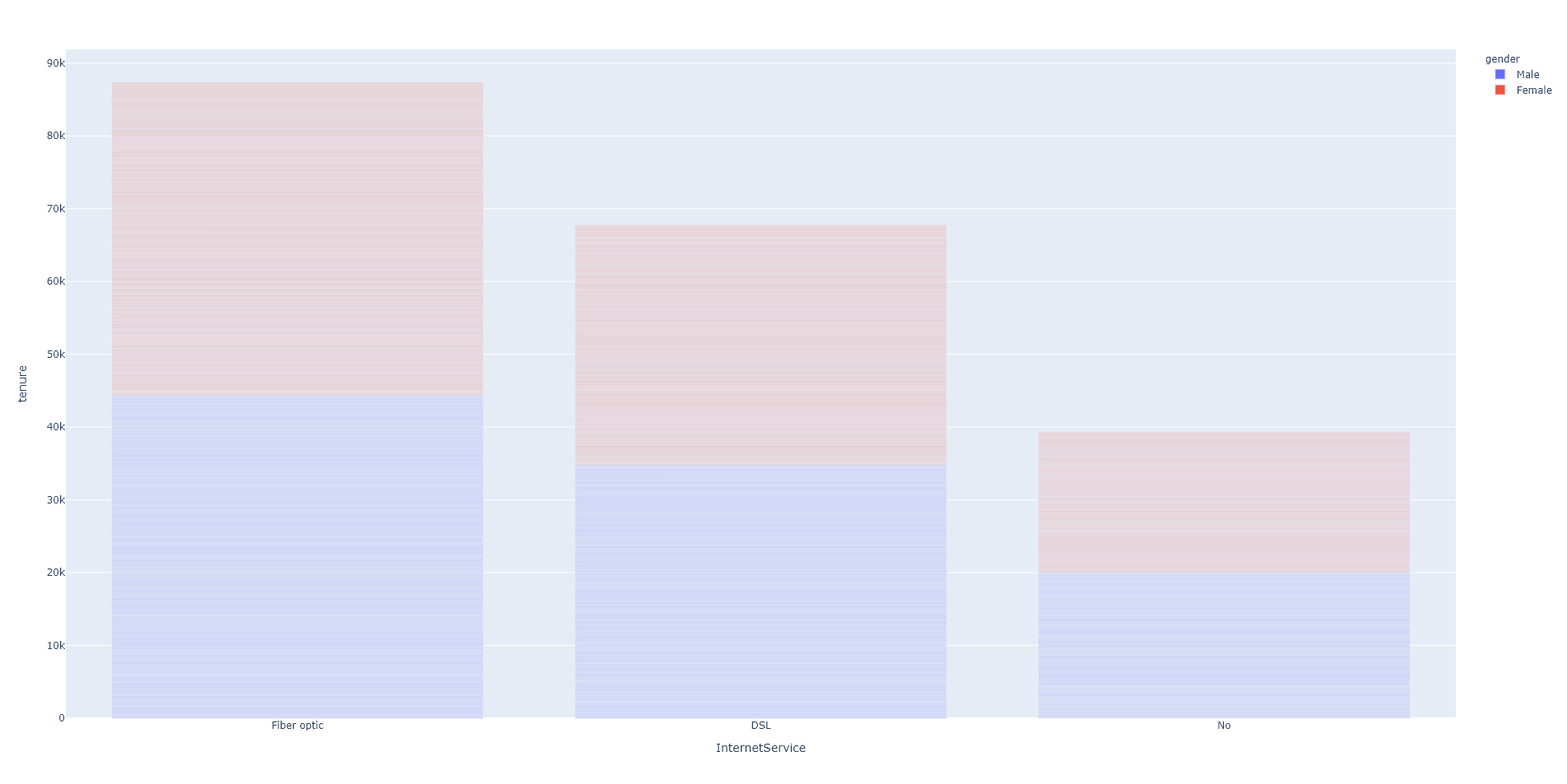Plotly Bar Plot - Tutorial and Examples - Stack Abuse

# Plotly Bar Plot - Tutorial and Examples### Introduction

Plotly is a JavaScript-based, Python data visualization library, focused on interactive and web-based visualizations. It has the simplicity of Seaborn, with a high-level API, but also the interactivity of Bokeh.

In addition to the core library's functionality, using the built-in Plotly Express with Dash, makes it an amazing choice for web-based applications and interactive, data-driven dashboards, usually written in Flask.

In this guide, we'll take a look at how to plot a Bar Plot with Plotly.

Bar charts are most commonly used to visualize categorical data, where the height of each bar represents the number of occurrences in that category.

### Plot a Bar Plot with Plotly

To plot a Bar Plot in Plotly, you simply call the bar() function of the Plotly Express (px) instance, providing the x and y arguments with valid data:

import plotly.express as px

x = ['Category 1', 'Category 2', 'Category 3']
y = [5, 3, 6]

fig = px.bar(x, y)
fig.show()


Here, we have three categories, as a list that we've provided to the x argument and a few scalar values we've provided to the y argument. This results in a simple and intuitive Bar Plot:However, we rarely work with lists when doing Data Visualization. Let's import the Telecom Users Dataset and visualize the InternetService and tenure features through a Bar Plot.

The InternetService feature is a categorical feature, that specifies which type of service the customer is using, while the tenure is a numerical feature that represents how long a customer has been with the company, in months:

import pandas as pd
import plotly.express as px

df = pd.read_csv('telecom_users.csv')
print(df.head())


This results in:

   gender  SeniorCitizen  tenure InternetService ...
0    Male              0      72              No ...
1  Female              0      44     Fiber optic ...
2  Female              1      38     Fiber optic ...
3    Male              0       4             DSL ...
4    Male              0       2             DSL ...


Now, let's go ahead and plot this data as a Bar Plot:

import pandas as pd
import plotly.express as px

df = pd.read_csv('telecom_users.csv')

fig = px.bar(df, x = 'InternetService', y = 'tenure')
fig.show()


To plot data from a dataset, we've provided the data source (df) as the first argument, and the column names we'd like to visualize to the x and y arguments. Plotly maps these, fetches the data and generates a plot:Now, the plot generated by Plotly actually separates each instance into a small stacked bar of its own on this plot, since several rows share the same x value, unlike the simple 1-to-1 mapping like we had in the first example.

We can see the cumulative number of months they've served to their customers, in parallel. While ~90K months may seem like an insane number of months (7500 years), the median tenure is 29 months:

print(df['tenure'].median()) # 29.0


### Change Bar Plot Colors with Plotly

It's easy to change the colors of each of the aforementioned instances, based on any other variable present in the dataset. These are most often other categorical features, such as gender or SeniorCitizen.

Grouping instances by another feature creates a Grouped Bar Plot, which are most commonly plotted one next to the other. By simply coloring instances based on another feature, we'll have a Stacked Grouped Bar Plot, since we'll have two or more groups of instances one on top of the other.

Let's take a look at the Bar Plot once again, once we color each plot depending on the gender feature:

import pandas as pd
import plotly.express as px

df = pd.read_csv('telecom_users.csv')

fig = px.bar(df, x = 'InternetService', y = 'tenure', color='gender')
fig.show()


Now, the default Plotly color-scheme will apply, and the instances will be sorted by color so they're not mish-mashed throughout the plot:Since we've got multiple rows sharing the same x values - these are plotted, essentially, as stacked grouped bars.

If we were to plot a different feature, that doesn't stack (isn't a binary choice of another feature), the plot would look fairly differently:

import pandas as pd
import plotly.express as px

df = pd.read_csv('telecom_users.csv')

fig = px.bar(df, x='tenure', y='MonthlyCharges', color='tenure')

fig.show()When providing many color values, such as 0..70 via the tenure feature - you'll see beautiful gradients formed in your plots.

### Plot Grouped Bar Plot with Plotly

Sometimes, it's easier to differentiate if we plot the bars one next to the other, instead of stacking them on top of each other. This is particularly useful when we have binary features, such as SeniorCitizen that only has two values. You can really have n values in features you group by, though, most groups don't really benefit us much if they're too large.

## Better understand your data with visualizations

•  30-day no-questions refunds
•  Beginner to Advanced
•  Updated regularly (update June 2021)
•  New bonus resources and guides

To do this, we can simply update the layout of the Figure, and set the barmode to 'group':

import pandas as pd
import plotly.express as px

df = pd.read_csv('telecom_users.csv')

fig = px.bar(df, x = 'InternetService', y = 'tenure', color='gender')
fig.update_layout(barmode='group')
fig.show()


Now, it's much easier to compare the number of instances:### Plot a Horizontal Bar Plot with Plotly

To plot a Bar Plot horizontally using Plotly, we can set the orientation argument to h (as opposed to the default v) while plotting the Bar Plot:

import pandas as pd
import plotly.express as px

df = pd.read_csv('telecom_users.csv')

fig = px.bar(df, x='MonthlyCharges', y='tenure', color='tenure', orientation='h')

fig.show()


Keep in mind that if you'd like to keep the X-Y relationship as it was before, you'll switch the x and y arguments. Running this code results in:### Ordering Bar Plots in Plotly

Plotly automatically orders the bars. You can directly modify this setting by updating the Figure parameters.

You can opt for manual ordering, or automatic ordering. For manual ordering, you can pass in a dictionary that contains the names of the features and their order, which will result in a fixed order, to the category_orders argument:

import pandas as pd
import plotly.express as px

df = pd.read_csv('telecom_users.csv')

fig = px.bar(df, x='InternetService', y='tenure', color='gender',
category_orders={"InternetService": ["DSL", "Fiber optic", "No"],
"gender": ["Female", "Male"]})
fig.show()


Here, we've ordered the InternetService feature in a specific order, that we've manually set, as well as the gender feature.

Plotting the Bar Plot now will result in:Alternatively, especially if there's a large number of possible values - we might want to let Plotly automatically sort and order values of features. We can either sort them categorically or numerically.

And both of these can further be ordered in ascending or descending order. To order numerically, we invoke the total keyword, while we invoke the category keyword for categorical ordering.

By default, Plotly adjusts the ordering based on the provided data - in our case, it ordered it as total ascending. Let's change that around to total descending:

fig.update_layout(xaxis={'categoryorder':'total descending'})


This results in:When ordering categorically, it sorts lexicographically:

fig.update_layout(xaxis={'categoryorder':'category ascending'})


This results in:### Conclusion

In this tutorial, we've taken a quick look at how to plot and customize Bar Plots with Plotly.

If you're interested in Data Visualization and don't know where to start, make sure to check out our bundle of books on Data Visualization in Python:

Data Visualization in Python with Matplotlib and Pandas is a book designed to take absolute beginners to Pandas and Matplotlib, with basic Python knowledge, and allow them to build a strong foundation for advanced work with theses libraries - from simple plots to animated 3D plots with interactive buttons.

It serves as an in-depth, guide that'll teach you everything you need to know about Pandas and Matplotlib, including how to construct plot types that aren't built into the library itself.

Data Visualization in Python, a book for beginner to intermediate Python developers, guides you through simple data manipulation with Pandas, cover core plotting libraries like Matplotlib and Seaborn, and show you how to take advantage of declarative and experimental libraries like Altair. More specifically, over the span of 11 chapters this book covers 9 Python libraries: Pandas, Matplotlib, Seaborn, Bokeh, Altair, Plotly, GGPlot, GeoPandas, and VisPy.

It serves as a unique, practical guide to Data Visualization, in a plethora of tools you might use in your career.

Last Updated: May 31st, 2021

## Improve your dev skills!

Get tutorials, guides, and dev jobs in your inbox.

No spam ever. Unsubscribe at any time. Read our Privacy Policy.

# Prepping for an interview?

• Improve your skills by solving one coding problem every day
• Get the solutions the next morning via email
• Practice on actual problems asked by top companies, like:

# Better understand your data with visualizations

•  30-day no-questions refunds
•  Beginner to Advanced
•  Updated regularly (update June 2021)
•  New bonus resources and guides

© 2013-2021 Stack Abuse. All rights reserved.